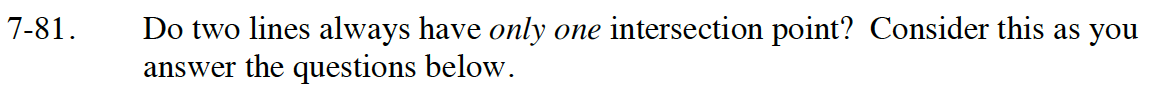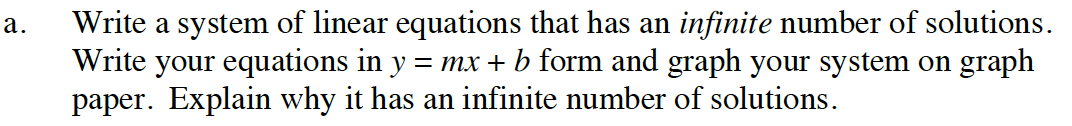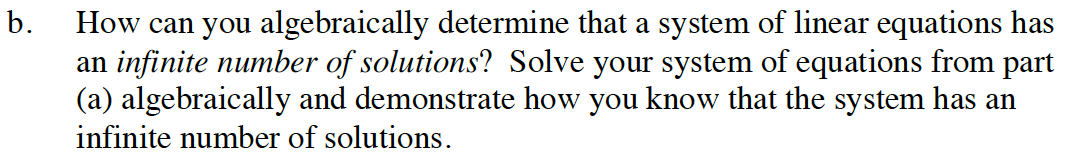Home > CC4 > Chapter 7 > Lesson 7.1.7 > Problem7-81

7-81.

Do two lines always have only one intersection point? Consider this as you answer the questions below. Homework Help ✎

1. Write a system of linear equations that has an infinite number of solutions. Write your equations in y = mx + b form and graph your system on graph paper. Explain why it has an infinite number of solutions.

2. How can you algebraically determine that a system of linear equations has an infinite number of solutions? Solve your system of equations from part (a) algebraically and demonstrate how you know that the system has an infinite number of solutionsWrite a system of equations using two lines that have the same slope and the same y-intercept.

Since the two lines have the same slope and the same y-intercept, the lines coincide (overlap).
So all of the points are solutions for both equations.Try solving 2x + 1 = 2x + 1 algebraically.

Subtracting 2x on both sides results in 1 = 1 which is a true statement. Therefore, there are an infinite number of solutions to this equation.